# Multi-Dimensional Data— boon or bane?

The ‘why, what & how’ of PCA (Principal Component Analysis)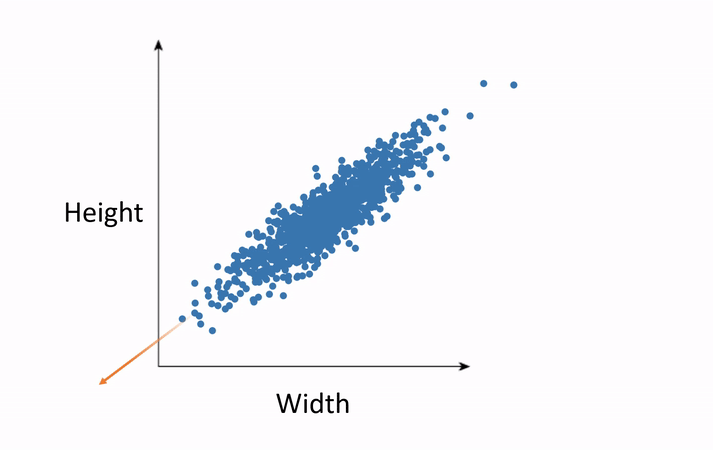Source: — megaputer.com

Availability of data needs no introduction. And, its needless to say, data drives businesses, these days. For a given business problem, the more features that we capture, the better the analysis. Isn’t it? Or is it?

What if, …

1. There are more dimensions/features than the number of observations?

2. There are too many dimensions, sometimes running into hundreds or more?

The above scenarios define a specific phenomenon called Curse of Dimensionality, which in simple words mean, too many features could actually be a problem. But, how?

Let’s say, we are trying to find out, if an employee will attrite or not. We have information like the employee’s age, education, experience, current job level, monthly income, etc. Here, we already know that, higher the age, more the experience; More the experience, higher the job level and higher monthly income. So, if we say, employees in the junior management are prone to attrite, it also means, employees with lesser experience might attrite. Correct? Similarly, percent salary hike and the performance rating would correlate; Job satisfaction and number of years in the same company might also correlate and so on. This correlation among the features (or independent variables), that is, a mutual relationship between the features , is called Multicollinearity.

The features (referring to the columns in a dataset), are also called as independent /predictor variables, using which the target/dependent/response variable is predicted. The target variable in the above example is “whether an employee will attrite or not — Attrition (Yes | No)”.

If some features convey the same information, could we just drop them as redundant? (keep just one column and drop the other redundant ones). In certain scenarios, yes, we can- where dropping them would not (hugely) impact the prediction of the model. Precisely, we are choose only the most relevant features. This is called, ‘Feature Selection’.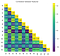Correlation heatmap

The image on the left shows the correlation of features (X1 to X13) in a sample data. Correlation coefficient ranges from -1 to +1, where the extreme values indicate maximum correlation and 0 indicates no correlation.

PS:- Correlation does not mean causation. eg. more sleep helps you perform better; More sleep and performance might correlate; when one increases, the other might also increase. But, more sleep does not cause (contribute to) better performance. The same can be applied to the above example.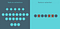Source: — quantdare.com/

For feature selection, we ought to know those features, that are inessential or those that contribute the least. It is also possible that, even after dropping them, multicollinearity may still exist. Well, what if, we can create a new set of derived variables that are a linear combination of the original variables? Such a technique is called ‘Feature Extraction’.

PCA (Principal Component Analysis) is an unsupervised algorithm used for feature extraction. The derived features are created in such a way that they are uncorrelated to each other, so that, each feature conveys unique information. While one of the purposes is to avoid redundancy in information, PCA also aims at reducing dimensions, that is, to reduce the number of features to be analyzed.

Here is an example of regression, where the value we are trying to predict is continuous (unlike the discrete labels in the above example). Eg. we would like to predict the price of houses (target variable) in a given locality. The features influencing this, could be, plot size, the pollution levels, accessibility, etc.

The original equation will be

House Price = w0 + w1 Plot size + w2 Pollution Level+ w3 Accessibility + ….

where, w1, w2, w3 — corresponding coefficients/weights for each feature. Keeping everything else constant, increase in one unit of Plot size, contributes to w1 times increase in House Price.

After, PCA, the same equation becomes

House Price= β0 + β1 PC1 + β2 PC2 + β3 PC3 + …

where,

PC1, PC2, PC3 — Principal components or new features

β1, β2, β3 — weights/coefficients of the principal components

With or without PCA, the predicted price do not vary much. However, without PCA, unit increase in one of the features might affect the weights of all the correlated variables and hence, it is not possible to identify feature importance — how much each feature contributes to the target variable. Explainability of the features is reduced.

# Concept of PCA: -

The principal components are constructed on the fact that the variance in the data is representative of the information in the data. A feature whose variance is zero, carries no information and it can be considered a constant.

In the above example, as the job levels increase, the monthly income increases. Let’s assume that there is no variance in monthly income. That way, monthly income would not vary for different job levels, and hence, it can be considered a constant, irrespective of the job levels.

It is due to this inherent variance present in the data, analysis can be done and information can be extracted.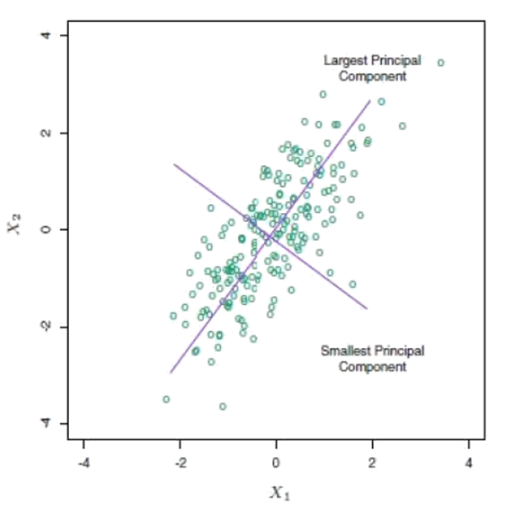Source: — docs.tibco.com

PCA captures maximum variance along the data. A dataset with n dimensions/features will result in n principal components. The component that captures the maximum variance is PC1 and the one with the smallest variance is PCn. The principal components are formed such that they are orthogonal to each other, that is they are uncorrelated (no linear relationship) and hence, each component carries useful information about the data. Since one of the goals is to reduce dimension, we choose k principal components, such that k < n and it captures at-least 70% and up-to 90% of the total variance in the data.

# Steps in PCA: -

1. Standardize features: -
2. Construct Covariance matrix: -
3. Eigen Decomposition: -
4. Sort eigen pairs: -
5. Choose optimum principal components: -

# 1. Standardize features: -

Since PCA captures the variance in the data, huge variance innate in a particular feature may be misleading.

Let’s say, age is between 30 to 60 years. However, monthly income may vary from, say INR 10K to about INR 2L . The variance in monthly income is significantly higher than that of age. If the features are not standardized, automatically, monthly income becomes the feature carrying most variance (among age & monthly income). It is also for the same reason that outliers are to be removed (or treated before the next steps). A person above 50 years in the junior management or someone at a younger age, becoming a CEO (hence, higher salary, etc.) are considered outliers in this data.

PCA becomes skewed towards features with large variance and the resulting principal components will not convey the original information.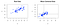Source: — cybernetist.com

Features are standardized using the z score, where the mean is subtracted from the observations, to center the data around the axis and then divided by the standard deviation so that they fall under the same scale.

where, x — observed value of the feature

µ — mean of the feature

σ — standard deviation around the mean

# 2. Covariance Matrix: -

A covariance matrix shows the interaction of features with one another. It tells us how much the variability in one feature affects the other.

where, Z — standardized data, ZT — transpose of the standardized data.

Why Covariance and not Correlation?: -

Covariance measures the direction of the linear relationship between two variables, either directly (positive) or inversely (negative) proportional. Correlation on the other hand, measures not only the direction, but also the strength of the linear relationship between two variables.

Covariance is an extension of variance, where instead of summation of the squared deviation of an observation from the mean, the sum-product of deviation of two variables from the respective means are considered. It is measured in square units and hence ranges between -∞ and +∞. A change in scale affects covariance. When the actual values are standardized, the covariance reduces to -1 < cov < 1.

Correlation is already standardized, because the calculation involves dividing covariance by the product of standard deviations of the variables. Correlation does not have any units and ranges between -1 to 1. Hence, correlation and covariance of standardized variables will be (approx.) the same.

COV(Scaled Data) = CORR(Scaled Data) = CORR(Unscaled Data)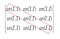Covariance Matrix

COV(X, Y) (in the image on the left) indicates how much X & Y vary together.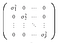Source: — analyticsvidhya.com

COV(X, Y) = COV(Y, X)

The diagonal of the covariance matrix indicates the variance of the feature — which is the covariance of the same feature with itself or the interaction of the feature with itself and is always one. Since the diagonal elements are always 1, the total variance (information) in the data = sum of the diagonal elements in a covariance matrix = number of variables/features/dimensions in the data.

# 3. Eigen Decomposition: -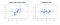Source: — cybernetist.com

The information in the original scaled and centered data, that was previously converted to the covariance matrix, is rotated around the axis to form the principal components (new dimensions) of the data. The principal components are also called as Eigen Vectors, which determines the direction of the maximum variance.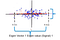Source: —analyticsvidhya.com

The maximum variance (x-max — x-min) also called as Signal (as shown in the image on the left) forms the direction of the largest variance and is taken as PC1. The signal indicates the amount of variance captured, also called as Eigen Values.

The residual variance (y-max — y-min) is called Noise. The signal to noise ratio (SNR) is given below. Better SNR indicates the ability to capture maximum variance (information in the data) and hence, results in a better model.

While the residual variance is categorized as noise for PC1, it holds information (variance) about the rest of the data and becomes the signal to the second principal component. All the n principal components, for the n features/dimensions in the data are constructed in the same way.

# 4. Sort Eigen pairs: -

Eigen vectors and eigen values always come in pairs, indicating the direction and the magnitude, respectively. The eigen values are to be sorted in descending order to obtain the highest to lowest variance and the respective eigen vectors gives the principal components.

Explained & Cumulative Variance: -

Explained Variance is the percentage of variability captured by each principal component. In the scree plot (plotted on sample data shown on the left), approximately 45% of the variability is captured by the first principal component.

Cumulative Variance is the total variance percentage captured from PC1 to the respective principal component. Cumulative variance captured by the last PC indicates the sum of variance % of all the PC, that is, it captures the total variance in the data, which is 100%.

# 5. Optimum Principal Components: -

Number of PCs chosen < Total dimensions (number of features). The idea is to explain a large amount of variance in the data by smaller number of PCs.

i. If k is the number of principal components chosen, then PC(k) is expected to capture at-least 70% and up-to 90% of the total variance in the data. However, this is subject to change with the data or the domain.

ii. Another way of deciding the number of principal components is to find the increase in the cumulative variance between two consecutive components. If the difference between the two cumulative variances of k and k+1 components, is less than 10%, then k components are taken, else, k+1 components are taken.

iii. Yet another way is to look for an elbow point in the “scree plot”, beyond which the line becomes approximately horizontal.

In the illustration above, for explaining approximately 70% variance, it is enough to choose four principal components. However, to explain 90% of the variance, seven principal components are to be chosen.

1. The original correlated variables (shown in the correlation heatmap above) is now converted to uncorrelated principal components.
2. The number of features are reduced from 13 to 7, each carrying unique information about the data.

The implementation of PCA for the Boston Housing price dataset in python (google colab), is available here.

# Final Thoughts: -

Having reduced the number of features, PCA contributes to a notable increase in model performance, given that the features are highly correlated. However, interpreting the principal components (derived features) is not straight forward. It is important to remember that the data has to be standardized before PCA. Care has to be taken to choose the correct number of principal components, so that there is an acceptable trade-off between the amount of information lost and the model accuracy.

While PCA comes to rescue, when the data has several dimensions, it’s equally important to restrict the data collection to relevant/optimal features, so that the resources are used efficiently. But, how much data (observations) is to be collected? That’s a story for another blog. Stay Tuned!

## Analytics Vidhya

Analytics Vidhya is a community of Analytics and Data…

## Analytics Vidhya

Analytics Vidhya is a community of Analytics and Data Science professionals. We are building the next-gen data science ecosystem https://www.analyticsvidhya.com

Written by

## Dhivya Karthic

Data Science Enthusiast | https://www.linkedin.com/in/dhivyakarthic/## Analytics Vidhya

Analytics Vidhya is a community of Analytics and Data Science professionals. We are building the next-gen data science ecosystem https://www.analyticsvidhya.com

Medium is an open platform where 170 million readers come to find insightful and dynamic thinking. Here, expert and undiscovered voices alike dive into the heart of any topic and bring new ideas to the surface. Learn more

Follow the writers, publications, and topics that matter to you, and you’ll see them on your homepage and in your inbox. Explore

If you have a story to tell, knowledge to share, or a perspective to offer — welcome home. It’s easy and free to post your thinking on any topic. Write on Medium